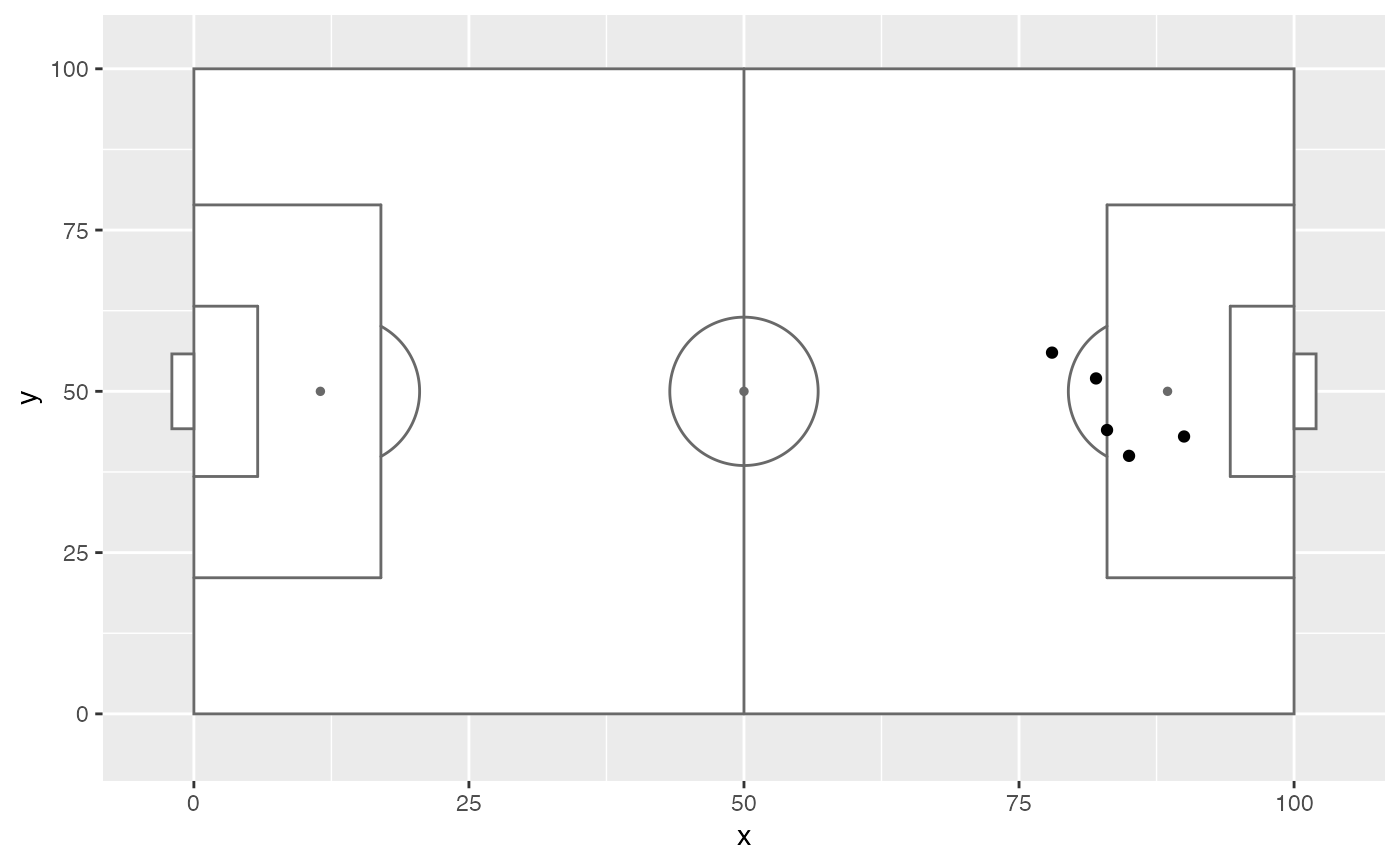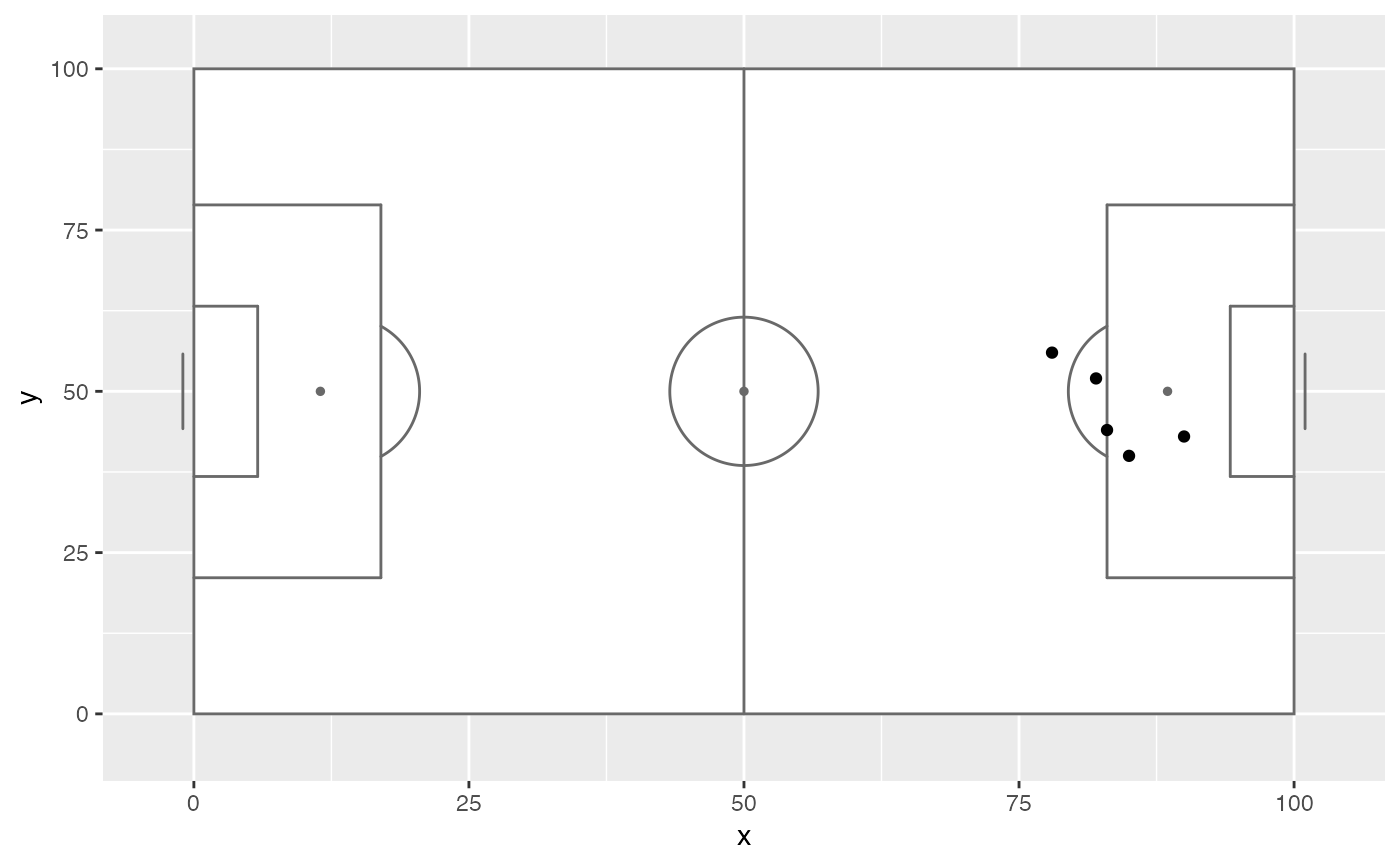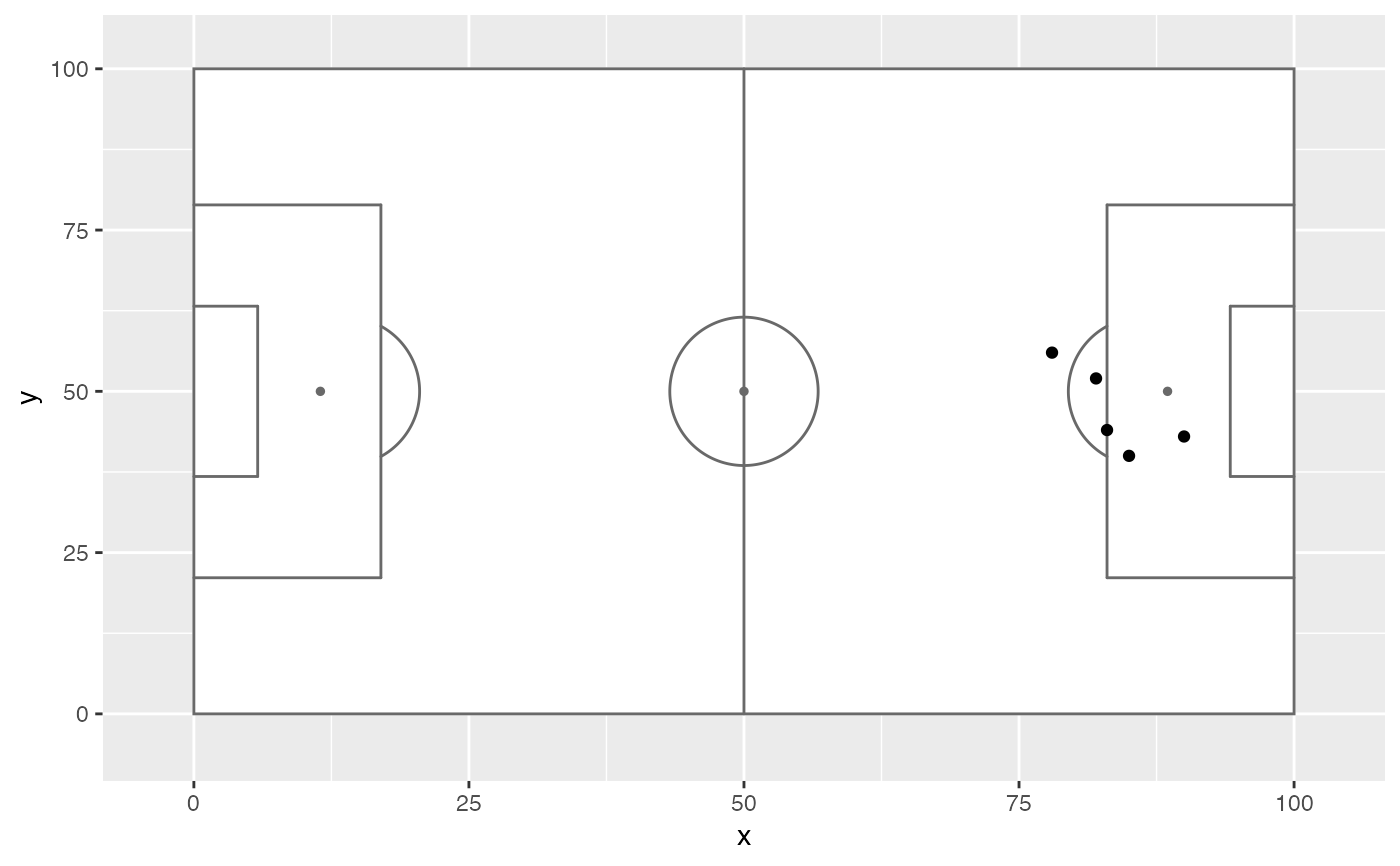Various functions ca be supplied to annotate_pitch to specify the appearance of goals in the resulting plot.

goals_box(colour, fill, dimensions, offset = 2, ...)

goals_strip(
colour,
fill,
dimensions,
offset = 1,
size = 1,
lineend = "round",
...
)

goals_line(colour, fill, dimensions, ...)

Arguments

colour

Colour of pitch outline.

fill

Colour of pitch fill.

dimensions

A list containing the pitch dimensions to draw. See help(pitch_opta).

offset

Determines how deep the goal extends.

...

Passed onto underlying ggplot2::annotate calls.

size

Determines line thickness in goals_strip and goals_line.

lineend

Determines lineend in goals_strip and goals_line.

Value

list of ggplot geoms to be added to a ggplot plot

Details

Each function takes colour, fill, and dimensions arguments. User-defined functions with the same arguments can also be used

Examples

shots_data <- data.frame(x = c(90, 85, 82, 78, 83),
y = c(43, 40, 52, 56, 44))

# Default
ggplot(shots_data, aes(x = x, y = y)) +
annotate_pitch(goals = goals_box) +# Other goals markings
ggplot(shots_data, aes(x = x, y = y)) +
annotate_pitch(goals = goals_strip) +# Partial functions can be used to customise further
ggplot(shots_data, aes(x = x, y = y)) +
annotate_pitch(goals = ~ goals_strip(..., size = 2)) +